International
Tables for
Crystallography
Volume C
Mathematical, physical and chemical tables
Edited by E. Prince

International Tables for Crystallography (2006). Vol. C, ch. 4.1, pp. 186-187

Section 4.1.2. Electromagnetic waves and particles

V. Valvodaa

aDepartment of Physics of Semiconductors, Faculty of Mathematics and Physics, Charles University, Ke Karlovu 5, 121 16 Praha 2, Czech Republic

4.1.2. Electromagnetic waves and particles

| top | pdf |

Both electromagnetic waves and particles can be described by the wavefunction ψ(r), as a complex function of spatial coordinates, by the wavelength λ, the wavevector k, which indicates the direction of propagation and is of magnitude 2π/λ, the frequency ν or angular frequencyin rad  s−1, and the phase velocity v (and the group velocity). Intensity in r is given by |ψ(r)|2. These wavefunctions are solutions of the same type of differential equation [see, for example, Cowley (1975)]: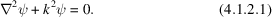For electromagnetic waves,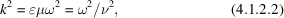where k is the wavenumber,is the permittivity or dielectric constant and μ is the magnetic permeability of the medium;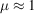for most cases. The velocity of the waves in free space is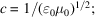otherwise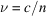, where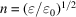is the refraction index.

For particles of mass m and charge q with kinetic energy Ek in field-free space, the wave equation (4.1.2.1)is the time-independent Schrödinger equation and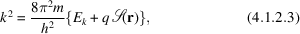where(r) is the electrostatic potential function and the bracket gives the sum of the kinetic and potential energies of the particles.

Important nontrivial solutions of (4.1.2.1)are (after adding the time dependence) the plane wavefunctions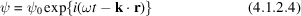or the spherical wavefunctions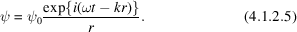Thus, relatively simple semi-classical wave mechanics, rather than full quantum mechanics, is needed for interactions with no appreciable loss of energy. The interaction of the waves with matter depends on the spatial variation of the refractive index given by the spatial variations of the electron density or the electrostatic potential functions.

Electromagnetic waves can also be described in terms of energy quanta, photons, with energy given by Planck's law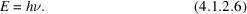The values of E, ν, and λ of the electromagnetic waves used in general crystallography are scaled in Fig. 4.1.2.1. It should be noted that there are several types of electromagnetic waves in the most important wavelength range near 1 Å, which are called X-rays (when generated in X-ray tubes), γ-rays (when emitted by radioactive isotopes) or synchrotron radiation (emitted by electrons moving in a circular orbit).Figure 4.1.2.1 | top | pdf |Comparison of the energy, frequency, and wavelength of the electromagnetic waves used in crystallography (logarithmic scale).

On the other hand, the beam of particles of mass m, moving with velocity v, behaves like waves with wavelength given by de Broglie's law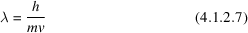or using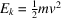for the kinetic energy of particles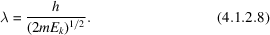When relativistic effects are taken into account,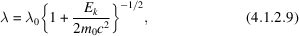where m0 is the rest mass and λ0 the non-relativistic wavelength. High-energy electrons (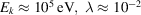Å) and neutrons (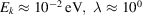Å) belong to the most prominent particles used in diffraction crystallography (see Table 4.1.3.1). However, low-energy electrons (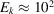eV,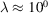Å), protons or ions of elements with quite high atomic number and energy (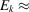103–106 eV) are also used in scattering, channelling or shadowing experiments (see Section 4.1.5).

 Table 4.1.3.1| top | pdf | Average diffraction properties of X-rays, electrons, and neutrons
X-raysElectronsNeutrons
(1) Charge 0 −1 e 0
(2) Rest mass 0 9.11 × 10−31 kg 1.67 × 10−27 kg
(3) Energy 10 keV 100 keV 0.03 eV
(4) Wavelength 1.5 Å 0.04 Å 1.2 Å
(5) Bragg angles Large Large
(6) Extinction length 10 µm 0.03 µm 100 µm
(7) Absorption length 100 µm 1 µm 5 cm
(8) Width of rocking curve 5′′ 0.6° 0.5′′
(9) Refractive index n1 n1 n ≶ 1
n = 1 + δ δ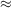− 1 × 10−5 δ+1 × 10−4 δ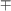1 × 10−6
(10) Atomic scattering amplitudes f 10−3 Å 10 Å 10−4 Å
(11) Dependence of f on the atomic number Z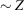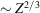Nonmonotonic
(12) Anomalous dispersion Common Rare
(13) Spectral breadth 1 eV 3 eV 500 eV
Δλ/λ10−4 Δλ/λ10−5 Δλ/λ2

References

Cowley, J. M. (1975). Diffraction physics, Chap. 1. Amsterdam: North-Holland.